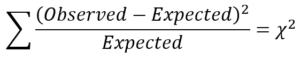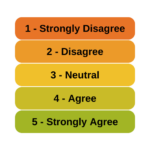# OptinMon 11 - Choose the Right Statistical Analysis Using Four Key Questions

### Six Common Types of Statistical Contrasts

September 18th, 2023 by

When you learned analysis of variance (ANOVA), it’s likely that the emphasis was on the ANOVA table, with its Sums of Squares and F tests, followed by a post-hoc test. But ANOVA is quite flexible in how it can compare means. A large part of that flexibility comes from its ability to perform many types of statistical contrast.

That F test  can tell you if there is evidence your categories are different from each other, which is a start. It is, however, only a start. Once you know at least some categories’ means are different, your next question is “How are they different?” This is what a statistical contrast can tell you.

### What is a Statistical Contrast?

A statistical contrast is a comparison of a combination of the means of two or more categories. In practice, they are usually performed as a follow up to the ANOVA F test. Most statistical programs include contrasts as an optional part of ANOVA analysis. (more…)

### What is Analysis of Means?

August 21st, 2023 by

The classic way to compare means in analysis of variance is examining pairwise differences in means after an F test.. It’s great for many problems, but sometimes your research question isn’t about pairwise differences.

Pairwise differences are not ideal if your research question is if, like the Sesame Street song, one of these groups is not like the others. Perhaps, you are trying to assure that groups conform to a common standard. Analysis of Means (ANOM) helps in all these settings. (more…)

### The Difference between Chi Square Tests of Independence and Homogeneity

October 14th, 2022 by

A chi square test is often applied to two-way tables, like the one below.

This table represents a sample of 1,322 individuals. Of these individuals, 687 are male, and 635 are female. Also 143 are union members, 159 are represented by unions, and 1,020 are not affiliated with a union.

You might use a chi-square test if you want to learn something about the relationship of gender and union status. The question then might come up: should you use a test of independence, or a test of homogeneity?

Does it matter? Software doesn’t generally differentiate between the two, which leads to a final question: are they even different?

Well, yes and no. Read on!

### Different: Independence versus Homogeneity

Independence and homogeneity do refer to different ideas. If union status and gender are independent, that means that union status and gender are unrelated. In other words, if you know someone’s union status, you won’t be able to make a better guess as to their gender.

If you know someone’s gender, you won’t be able to make a better guess as to their union status.

Homogeneity is different and refers to the concept of similarity. If you are familiar with linear regression, you might associate this with residuals. Residuals should be homogeneous, meaning they all come from the same distribution.

That idea applies to this two-way table as well. We may want to know if the distribution of union status is the same for men and women. In other words, does union status come from the same distribution for both men and women?

To test independence, we would not approach the question from the standpoint of gender or union status. We would take a sample of all employed individuals, and then break them down into the categories in the table.

To test homogeneity, we would approach it from the standpoint of gender. We would randomly sample individuals from within each gender, and then measure their union status.

Either approach would result in the table above.

### Same: Chi-Square Statistics

Chi-square statistics for categorical data generally follow this formula:For each of the six cells representing a combination of gender and union status, the number in the cell is the count we observe. “Expected” refers to what we would see in each cell under the null hypothesis. That means if gender and union status are independent (or if union status is homogeneous across the genders).

We calculate the difference, square it, and divide by the expected count for each cell. We then add these all together, and that is the chi-square test statistic.

Where do we get the expected counts for each cell?

Let’s examine the combination of male and union member under independence. If gender and union membership are independent, then how many male union members do we expect? Well,
– 10.81% of the sample are union members
– 51.96% are male

So, if they are independent, 10.81% x 51.96% is 5.62%, and 5.62% of 1,322 is 74.3. This is how many individuals we would expect to be male union members.

Now let’s consider male union members under homogeneity. Overall, 10.81% of the sample are union members. If this is the same for both males and females, then of the 687 males, we expect 74.3 to be union members.

Independence and homogeneity result in the same expected number of union members! It turns out this calculation is the same for every cell in the table. It follows that the chi-square statistic is also the same.

### Does It Matter?

As it turns out, independence and homogeneity are two sides of the same coin. If gender and union status are independent, then union status is distributed the same way for males and females.

So which test should you say you are using, if they turn out the same?

Again, that comes back to how you have phrased your research question. Are you determining whether gender and union status are related. That is a test of independence. Are you looking for differences between males and females? That is a test of homogeneity.

### Member Training: Analyzing Likert Scale Data

August 31st, 2022 byIs it really ok to treat Likert items as continuous?And can you just decide to combine Likert items to make a scale? Likert-type data is extremely common—and so are questions like these about how to analyze it appropriately. (more…)

### What Is Specification Error in Statistical Models?

June 8th, 2022 by

When we think about model assumptions, we tend to focus on assumptions like independence, normality, and constant variance. The other big assumption, which is harder to see or test, is that there is no specification error. The assumption of linearity is part of this, but it’s actually a bigger assumption.

What is this assumption of no specification error? (more…)

### Six Easy Ways to Complicate Your Analysis

July 13th, 2021 by

It’s easy to make things complex without meaning to. Especially in statistical analysis.

Sometimes that complexity is unavoidable. You have ethical and practical constraints on your study design and variable measurement. Or the data just don’t behave as you expected. Or the only research question of interest is one that demands many variables.

But sometimes it isn’t. Seemingly innocuous decisions lead to complicated analyses. These decisions occur early in the design, research questions, or variable choice.